## Example Questions

← Previous 1

### Example Question #1 : How To Square A Decimal

Which quantity is greater? When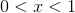Quantity AQuantity B

1

Quantity B is greater.

The two quantities are equal.

Quantity A is greater.

The relationship cannot be determined from the information given.

Quantity B is greater.

Explanation:

X must equal a number between 0 and 1.

If you square any decimal number in this range you will get an answer less than 1.  Try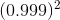= 0.998.

Therefore Quantity B is always larger.

### Example Question #2 : How To Square A Decimal

Which is greater, whenQuantity AQuantity B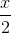Quantity A is greater

The relationship cannot be determined from the information given

Quantity B is greater

The two quantities are equal

The relationship cannot be determined from the information given

Explanation:

Whenmust be a decimal. Therefore it will decrease when it is squared. To find this answer we can substitute two values for.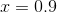and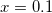When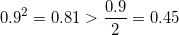But when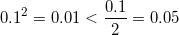Therefore we cannot tell which is greater based on the information given.

### Example Question #3 : How To Square A Decimal

Which is greater, whenQuantity AQuantity B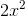Quantity B is greater

The relationship cannot be determined from the information given

The two quantities are equal

Quantity A is greater

The relationship cannot be determined from the information given

Explanation:

Whenmust be a decimal. Therefore it will decrease when it is squared. To find this answer we can substitute two values for.andWhen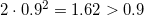But when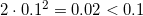Therefore we cannot tell which is greater based on the information given.

### Example Question #4 : How To Square A Decimal

Which is greater, when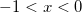Quantity A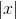Quantity BThe relationship cannot be determined from the information given

Quantity B is greater

The two quantities are equal

Quantity A is greater

Quantity A is greater

Explanation:

Whenmust be a negative decimal. Therefore it will decrease when it is squared and become positive. The first quantityis the distance to 0 from, in other words the positive value of. The second quantitywill always be positive, but because it is a decimal it will always be less than, ifwas a positive quantity. Since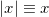, thenwiill always be greater than.

Quantity A is greater.

### Example Question #5 : How To Square A Decimal

Which is greater, whenQuantity AQuantity BQuantity A is greater

The two quantities are equal

The relationship cannot be determined from the information given

Quantity B is greater

Quantity B is greater

Explanation:

Whenmust be a negative decimal. Therefore it will decrease when it is squared and become positive. Becausemust be a decimal,will also be a decimal. Thereforewill never be greater than 1.

Quantity B is greater.

### Example Question #1 : Basic Squaring / Square Roots

Find the square root of the following decimal: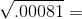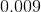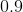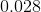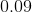Explanation:

The easiest way to find the square root of a fraction is to convert it into scientific notation.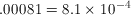The key is that the exponent in scientific notation has to be even for a square root because the square root of an exponent is diving it by two. The square root of 9 is 3, so the square root of 8.1 is a little bit less than 3, around 2.8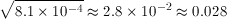### Example Question #2 : How To Find The Square Root Of A Decimal

Find the square root of the following decimal: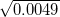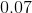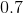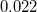Explanation:

To find the square root of this decimal we convert it into scientific notation.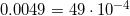Because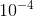has an even exponent, we can divide the exponenet by 2 to get its square root.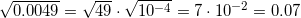### Example Question #3 : How To Find The Square Root Of A Decimal

Find the square root of the following decimal: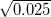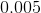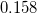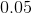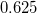Explanation:

This problem can be solve more easily by rewriting the decimal into scientific notation.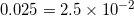Because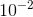has an even exponent, we can take the square root of it by dividing it by 2. The square root of 4 is 2, and the square root of 1 is 1, so the square root of 2.5 is less than 2 and greater than 1.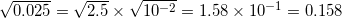### Example Question #4 : How To Find The Square Root Of A Decimal

Find the square root of the following decimal: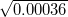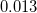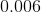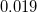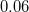Explanation:

This problem becomes much simpler if we rewrite the decimal in scientific notation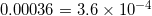Becausehas an even exponent, we can take its square root by dividing it by two. The square root of 4 is 2, and because 3.6 is a little smaller than 4, its square root is a little smaller than 2, around 1.9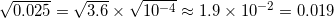### Example Question #5 : How To Find The Square Root Of A Decimal

Find the square root of the following decimal: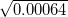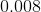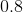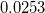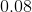Explanation:

To find the square root of this decimal we convert it into scientific notation.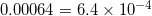Becausehas an even exponent, we can divide the exponenet by 2 to get its square root. The square root of 9 is 3, and the square root of 4 is two, so the square root of 6.4 is between 3 and 2, around 2.53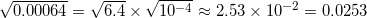← Previous 1

Tired of practice problems?

Try live online GRE prep today.